# Ray method

A method for formally finding high-frequency asymptotic approximations of solutions of problems in the theory of diffraction and propagation of waves. The ray method consists of an aggregate of methods of finding various versions of geometric approximation to the solution of the corresponding problem.

Suppose, for example, that the wave phenomenon is described by the wave equation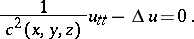If one adopts a geometric approximation for the wave equation and equates to zero the coefficients of successive powers of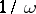(the parametercorresponds to the oscillation frequency), one arrives at the relationsThe first of these is the eikonal equation, and the remaining equations are called the transport equations. This is a recursive sequence of linear equations along rays, that is, extremals of the Fermat functional (see Fermat principle).

If one is given a surface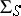on which the classical homogeneous boundary conditions are satisfied and if the vector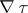is not tangent to, then for a given incident geometric approximation of the wave field one can find a reflected, or reflected and refracted, geometric approximation, which together formally satisfy the boundary conditions. Suppose, for example, that the Dirichlet boundary condition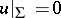is satisfied on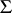; then a reflected geometric approximation is sought in the form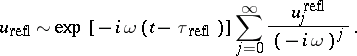(1)

Formal fulfillment of the boundary condition leads to the equalities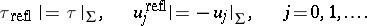The first condition implies the classical law of reflection of rays: "the angle of incidence is equal to the angle of reflection" . The equalities for the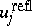specify initial data for the transport equations, which thesatisfy. Thus, the specification of an "incident wave" completely determines the reflected wave (1). One similarly considers the problem of refraction on the interface between two media.

In a neighbourhood of a singularity of a field of rays geometric approximation cannot be applied and one must use more complicated expansions, for example some unique asymptotic approximation or some version of the boundary-layer method (the most important version of the latter is apparently the parabolic-equation method).

The ray method complemented in this way makes is possible to construct formal high-frequency asymptotic expansions for a sufficiently-wide class of diffraction problems. Apparently, in practice such expansions are always asymptotic expansions of the solutions. This assertion has not yet been proved in such generality; its truth has been established only for special cases (when one either constructs an explicit solution or obtains an estimate, uniform in, of the norm of the operator inverse to the wave operator under the conditions in question). A one-dimensional version of the ray method is the WKB method; the version in quantum mechanics is semi-classical approximation. The ray method can be used successfully in the study of high-frequency waves of any kind (for example, electromagnetic). A more remote analogue of the ray method is the ray method for Rayleigh waves propagating along the surface of an elastic body of arbitrary shape, for waves on the surface of a heavy liquid, for weakly-curved elastic or acoustic layers, etc.

One of the generalizations is obtained if the geometric approximation is replaced by a series: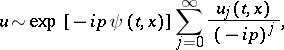(2)

whereis an expansion parameter. The theory of such expansions is called space-time geometrical optics, or the theory of waves modulated in both frequency and amplitude (see ). Expansions of the form (2) can also be used in the case when the equations that describe the wave phenomenon are no longer differential equations in time (as for dispersive media). The expansions (2) are used in the case of both differential and pseudo-differential equations. The mathematical techniques for constructing parametrices (in the case of hyperbolic equations) in the theory of Fourier integral operators (cf. Fourier integral operator) involve some version of space-time geometrical optics (cf. ). Non-linear analogues have been developed as well (see ).

Other versions of the ray method are connected with the analysis of discontinuous equations: in many situations discontinuities are described by means of a non-stationary version of the ray method and propagate along rays. The non-stationary analogue of the ray method is at the basis of the so-called Hadamard method for investigating the Cauchy problem for linear hyperbolic equations of the second order and its generalizations to systems of linear hyperbolic differential or pseudo-differential equations.